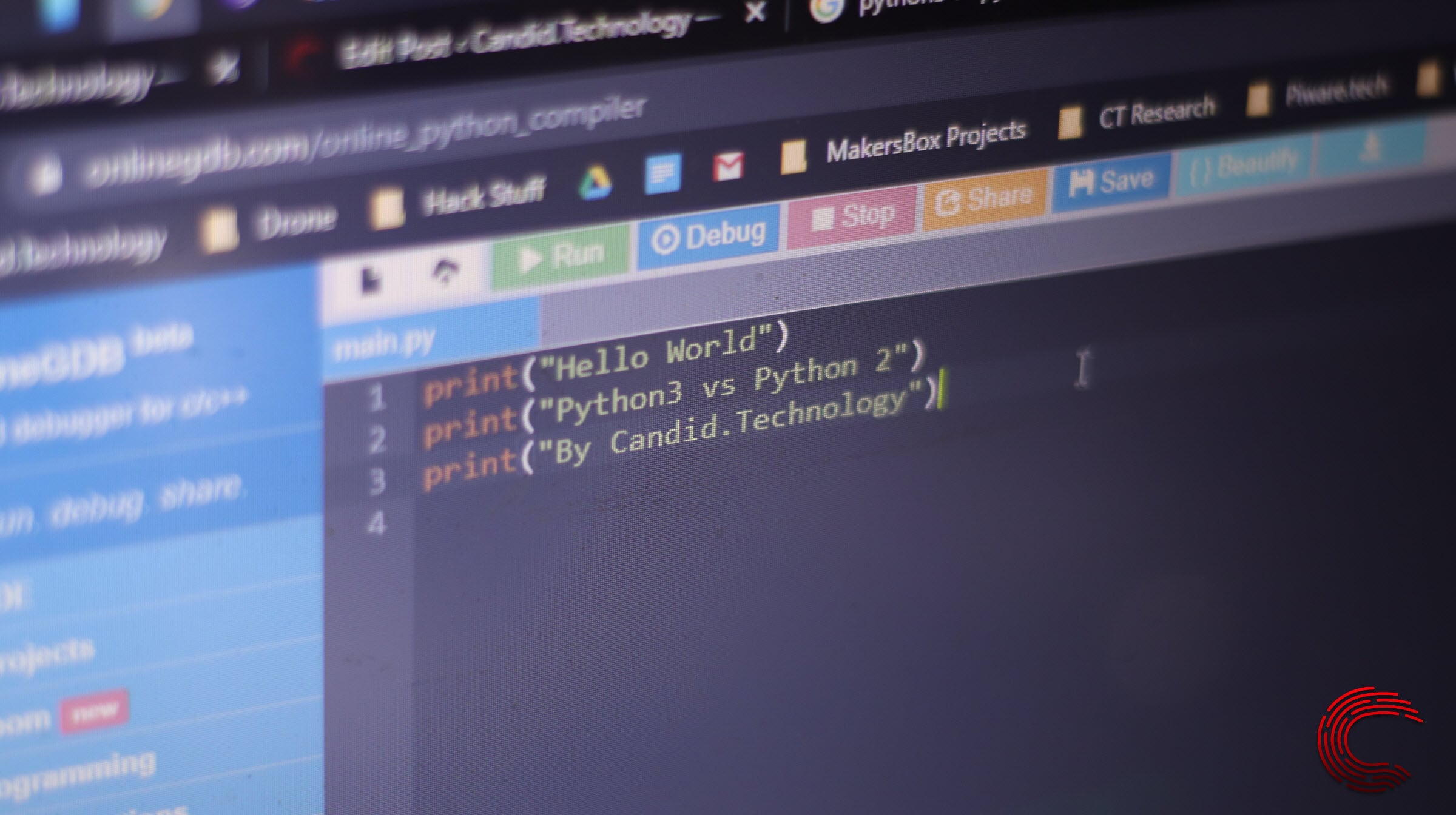# How to create a list in Python?Lists resemble dynamic arrays in many ways, except they don’t need to be homogeneous always, which is a huge advantage when you’re working in Python.

A single list can contain data types like Strings, Integers and Objects. Lists are mutable as well, which means they can be updated even after their creation.

In this article, we’re going to take a look at how you can create lists in Python.

# Creating a Python list

In Python, creating a list is as simple as placing a sequence inside the square brackets. Lists don’t require a built-in function for creation. You can even nest a list inside another list.

``list = [10, 20, 30, 40, 50]``

The above command creates a list containing five elements. You can create lists with different types of values.

``list = [ 'Candid', 10, 'Technology']``

You can make a list with recurring or duplicate values as well.

``list = [1, 2, 2, 3, 3, 3]``

Both the lists above are perfectly fine to create and use in Python.

## Fetching the size of a list

You can know the number of elements in a list by using the len() method.

``````list = [10, 20, 40]
print(len(list))``````

The above snippet will output 3, which is the size of the list.

Also read: How to install Python on Windows?

## Adding elements to a list

There are three ways of adding elements to a list.

• Using the append() method.
• Using the insert() method.
• Using the extent() method.

## Using the append() method

The append() method simply adds the specified element at the end of the list.

``````list = [10, 20, 30]
list.append(40)``````

The above snipped will add the value 40 at the end of the specified list.

## Using the insert() method

The insert() method allows you to add a value to any position in the list, as compared to the append() method, which only adds a value at the end. When using this function, you first specify the position of the element and then the element itself.

``````list = [10, 20, 30]
list.insert(2, 15)``````

After running the above snippet, the new list will be 10, 20, 15, 30.

Also read: How to use the Python range() function?

## Using the extend() method

The extend() method works just like the append() one, except it adds multiple elements at once to the end of a list.

``````list = [10, 20, 30]
list.extend([40, 50, 60])``````

The snippet above will add the elements 40, 50, 60 to the end of the specified list.

## Accessing a list’s elements

We use the index operator [ } to access elements from a list at a particular index. Do keep in mind that your index should be an integer.

``````list = [10, 20, 30]
print(list)``````

The aforementioned snippet will print 20 as that’s the element at the first index in the list.

Also read: How to concatenate strings in Python?